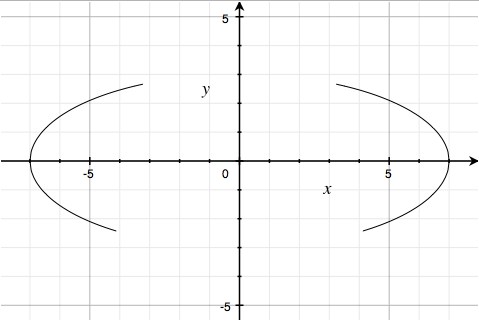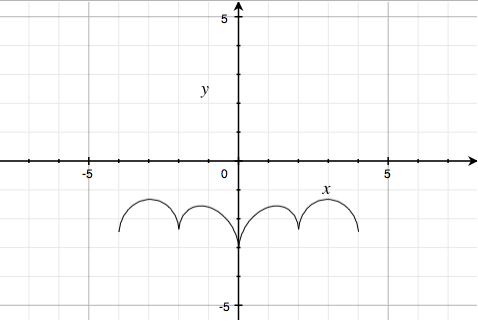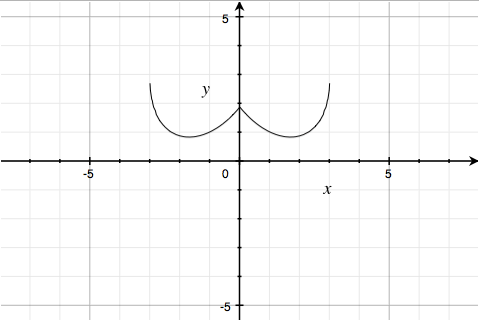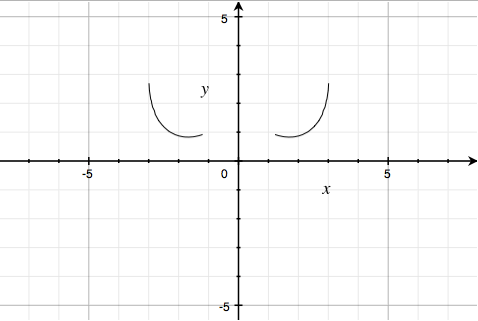## Posts Tagged ‘interesting math equations’

### The Batman Equation

August 2, 2011

Yes, the Batman Equation is for real.  I came across this on a friend’s Facebook posting and checked it out for myself.    I found the following explanation at Mathematics (in comment 187). Many students would find this really fun.

Explanation:

As Willie Wong observed, including an expression of the form|α|αis a way of ensuring thatα>0. (As|α|/α−−−−√is1ifα>0and non-real ifα<0.)

The ellipse(x7)2+(y3)2−1=0looks like this:So the curve(x7)2∣∣∣∣x∣∣−3∣∣∣∣x∣∣−3−−−−−√+(y3)2∣∣y+333√7∣∣y+333√7−−−−−−√−1=0is the above ellipse, in the region where|x|>3andy>−333−−√/7:That’s the first factor.

The second factor is quite ingeniously done. The curve∣∣x2∣∣−(333√−7)112×2−3+1−(||x|−2|−1)2−−−−−−−−−−−−−−√−y=0looks like:This is got by addingy=∣∣x2∣∣−(333√−7)112×2−3, a parabola on the positive-x side, reflected:andy=1−(||x|−2|−1)2−−−−−−−−−−−−−−√, the upper halves of the four circles(||x|−2|−1)2+y2=1:The third factor9(∣∣(1−∣∣x∣∣)(∣∣x∣∣−.75)∣∣)(1−∣∣x∣∣)(∣∣x∣∣−.75)−−−−−−−−−−−−√−8|x|−y=0is just the pair of lines y = 9 – 8|x|:truncated to the region0.75<|x|<1.

Similarly, the fourth factor3|x|+.75(∣∣(.75−∣∣x∣∣)(∣∣x∣∣−.5)∣∣(.75−∣∣x∣∣)(∣∣x∣∣−.5))−−−−−−−−−−−−−−√−y=0is the pair of linesy=3|x|+0.75:truncated to the region0.5<|x|<0.75.

The fifth factor2.25∣∣(.5−x)(x+.5)∣∣(.5−x)(x+.5)−−−−−−−−−√−y=0is the liney=2.25truncated to−0.5<x<0.5.

Finally,610√7+(1.5−.5|x|)−(610√)144−(|x|−1)2−−−−−−−−−−−√−y=0looks like:so the sixth factor610√7+(1.5−.5|x|)∣∣∣∣x∣∣−1∣∣∣∣x∣∣−1−−−−−√−(610√)144−(|x|−1)2−−−−−−−−−−−√−y=0looks likeAs a product of factors is0iff any one of them is0, multiplying these six factors puts the curves together, giving: (the software, Grapher.app, chokes a bit on the third factor, and entirely on the fourth)